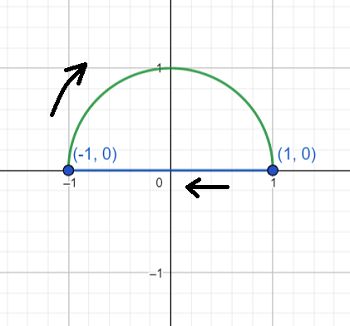# 7. Sometimes we can compute the line integral of a curve that is not closed by closing it and...

## Question:

7. Sometimes we can compute the line integral of a curve that is not closed by closing it and using Green's Theorem and then subtract away the work done on the curve that we used to close it. We do this because to compute the line integral of the original un-closed curve directly using

{eq}\int_{t=a}^{t=b} \vec{F}(\vec{r}(t)) \cdot \vec{r'}(t) {/eq} dt would be horrendous! Consider the problem below:

Let {eq}\vec{F}(x, y) {/eq} be a vector field in the plane given by the formula

{eq}\vec{F}(x,y)=(x^2-2xye^{-z^2}+2y_1e^{-x^2}+\frac{1}{\sqrt{y^2+1}}). {/eq}

If C is the path which goes from from (-1, 0) to (1, 0) along the semi circle {eq}x^2 + y^2 = 1, y \geq 0 {/eq}, evaluate{eq}\int_C \vec{F} \cdot d \vec{r}. {/eq}

## Green's Theorem:

Green's Theorem is one of the higher dimensional versions of the fundamental theorem of calculus (this is the one for double integrals) and is one of the most important results coming out of vector calculus. With it we can turn a closed path line integral into an area integral instead:

{eq}\begin{align*} \oint_C \vec F \cdot d\vec r****= \iint_D \left( \frac{\partial Q}{\partial x} - \frac{\partial P}{\partial y} \right) \ dA \end{align*} {/eq}

As mentioned, we can make use of Green's Theorem in the case for a non-closed curve by closing it off, and then subtracting the extra path.We can use Green's Theorem to get the closed path line integral, then subtract the linear (blue) path.

Assuming the field is supposed to be

{eq}\begin{align*} \vec{F}(x,y)= \left< x^2-2xye^{-x^2}+2y,\ e^{-x^2}+\frac{1}{\sqrt{y^2+1}} \right> \end{align*} {/eq}

the difference of the partials is

{eq}\begin{align*} \frac{\partial }{\partial x} \left( e^{-x^2}+\frac{1}{\sqrt{y^2+1}} \right) - \frac{\partial }{\partial y} \left( x^2-2xye^{-x^2}+2y \right) &= -2x e^{-x^2} + 2x e^{-x^2} - 2 \\ &= -2 \end{align*} {/eq}

We are negatively oriented, so we use Green's Theorem to write

{eq}\begin{align*} \int_C \vec F \cdot d\vec r &= - \iint_R (-2)\ dA \\ &= 2A \end{align*} {/eq}

And since the area enclosed is half the unit circle we have {eq}A = \frac\pi2 {/eq}, and so

{eq}\begin{align*} \int_C \vec F \cdot d\vec r &= \pi \end{align*} {/eq}

Now we need to get rid of the path along {eq}y = 0 {/eq}. So {eq}dy = 0 {/eq} and {eq}x {/eq} goes from 1 to -1. We find

{eq}\begin{align*} \int_{C_2} \left< x^2-2xye^{-x^2}+2y,\ e^{-x^2}+\frac{1}{\sqrt{y^2+1}} \right> \cdot d\vec r_2 &= \int_{1}^{-1} \left< x^2 - 0 + 0,\ e^{-x^2} + 1 \right> \cdot \left< dx, 0 \right> \\ &= - \int_{-1}^1 x^2\ dx \\ &= - \left[ \frac13 x^3 \right]_{-1}^1 \\ &= -\frac13 (1+1) \\ &= -\frac23 \end{align*} {/eq}

And so the line integral along the arc is

{eq}\begin{align*} \int_{C_1} \vec F \cdot d\vec r_1 &= \pi - \left( - \frac23 \right) \\ &= \pi + \frac23 \end{align*} {/eq}